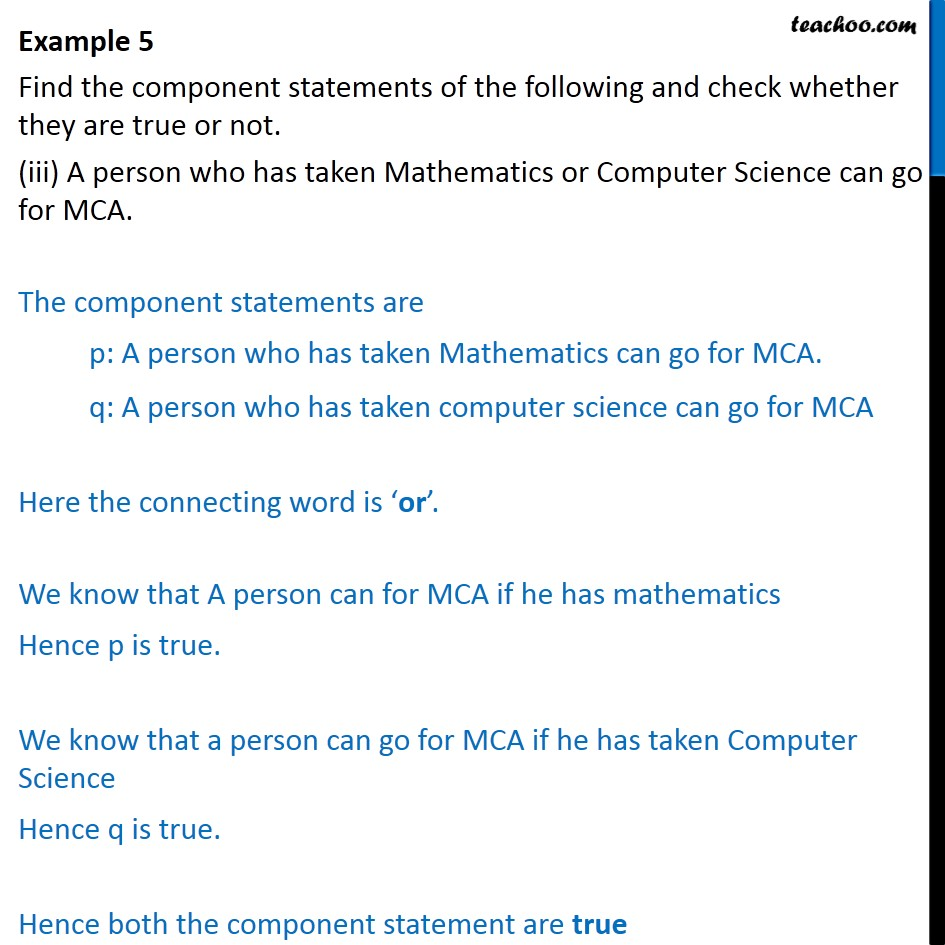1. Chapter 14 Class 11 Mathematical Reasoning (Deleted)
2. Serial order wise
3. Examples

Transcript

Example 5 Find the component statements of the following and check whether they are true or not. (iii) A person who has taken Mathematics or Computer Science can go for MCA. The component statements are p: A person who has taken Mathematics can go for MCA. q: A person who has taken computer science can go for MCA Here the connecting word is or . We know that A person can for MCA if he has mathematics Hence p is true. We know that a person can go for MCA if he has taken Computer Science Hence q is true. Hence both the component statement are true